# Transmission Lines

### What is Transmission Lines?

Transmission lines is the long conductor with special design to carry bulk amount of generated power at very high voltage from one station to another as per variation of the voltage level.Transmission lines

### Types of Transmission Line

In transmission line determination of voltage drop, transmission efficiency, line loss etc. are important things to design. These values are affect by line parameter R, L and C of the transmission line. Length wise transmission lines are three types.

### Short Transmission Line

• A length less than 80km (50 miles)
• Voltage level less than 69 kV
• Capacitance effect is negligible
• Only resistance and inductance are taken in calculation capacitance is neglected.

### Medium Transmission Line

A medium transmission line is classified as a transmission line with:

• A length more than 80 km (50 miles) but less than 250 km (150 miles)
• Operational voltage level is from 69 kV to approx 133 kV
• Capacitance effect is present
• Distribute capacitance form is use for calculation purpose.

### Long Transmission Line

A long transmission line is classified as a transmission line with:

• A length more than 250 km (150 miles)
• Voltage level is above 133 kV
• Line constants are consider as distribute over the length of the line

### Efficiency of Transmission Line

Transmission efficiency is define as the ration of receiving end power PR to the sending end power PS and it is express in percentage value.

cosθs is the sending end power factor.
cosθR is the receiving end power factor.
Vs is the sending end voltage per phase.
VR is the receiving end voltage per phase.

### Voltage Regulation of Transmission Line

Voltage regulation of transmission line is define as the ratio of difference between sending and receiving end voltage to receiving end voltage of a transmission line between conditions of no load and full load. It is also express in percentage.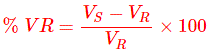Where, Vs is the sending end voltage per phase and VR is the receiving end voltage per phase.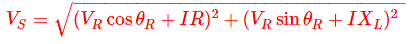XL is the reactance per phase.

R is the resistance per phase.
cosθR is the receiving end power factor.
Effect of load power factor on regulation of transmission line:

1. For lagging load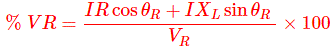2. For leading load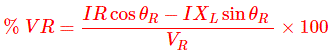Now

• Power factor is lagging or unity, and then VR is increased and goes to be positive.
• Power factor is leading, and then VR is decreased and goes to be negative.

### Load Power Factor on Efficiency of Transmission Line

We know efficiency of transmission line is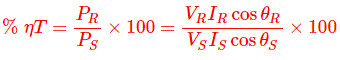Now, for short transmission line, IR = IS = I
So, considering three phase short transmission line,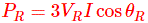So,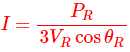Now it is clear that to transmit given amount of power, the load current is inversely proportional to receiving end power factor.

Again in case of medium and long transmission line,

Here it is clear that transmission efficiency depends on the receiving end power factor.

### Nominal T Method in Medium Transmission Line

In the nominal T method the capacitance of the line is assumed to be concentrated at the middle point of the line, and at both side half of line resistance and inductance is lumped.

Here,
IR is the receiving end load current per phase,
R is the resistance per phase,
XL is the inductive reactance per phase,
C is the capacitance per phase,
cosΦR is the receiving end lagging power factor,
VS is the sending end voltage.
V1 is the voltage across the capacitor.

Voltage across Capacitor C,

Capacitive current

Sending end current

Sending end voltage

### Nominal π Method in Medium Transmission Line

In the nominal pi method total line capacitance is assumed to be lumped and divided into two halves to be connected across sending end and receiving end respectively. Total line resistance and inductance are assumed to be present in middle of the line.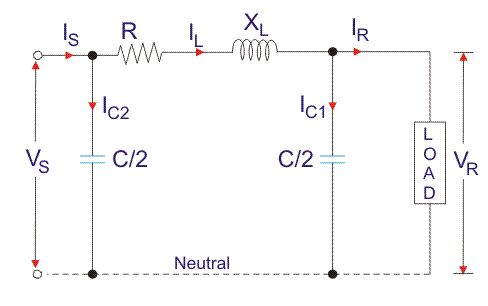Nominal π Method in Medium Transmission Line

Here IR is the receiving end load current per phase,
R is the resistance per phase,
XL is the inductive reactance per phase,
C is the capacitance per phase,
cosΦR is the receiving end lagging power factor,
VS is the sending end voltage.
Let us assume,

as the reference phasor,

Load current at receiving end

The capacitive current at load end

Line current

Sending end voltage,

Charging current at the sending end is

Sending End current is

### What is Nominal T Method in Medium Transmission Line?

In this method the whole line capacitance is assumed to be concentrated at the middle point of the line and half the line resistance and reactance are lumped on its either side. Therefore in this arrangement full charging current flows over half the line.